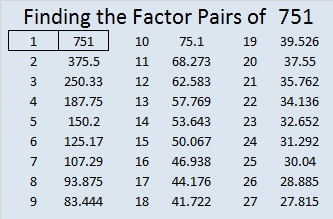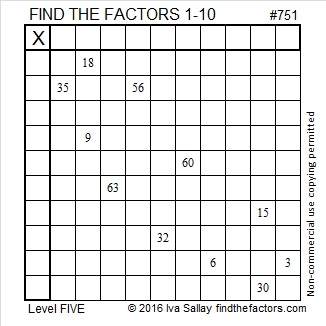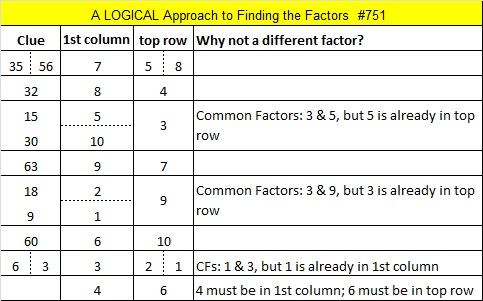# 751 and Level 5

• 751 is a prime number.
• Prime factorization: 751 is prime.
• The exponent of prime number 751 is 1. Adding 1 to that exponent we get (1 + 1) = 2. Therefore 751 has exactly 2 factors.
• Factors of 751: 1, 751
• Factor pairs: 751 = 1 x 751
• 751 has no square factors that allow its square root to be simplified. √751 ≈ 27.404379.How do we know that 751 is a prime number? If 751 were not a prime number, then it would be divisible by at least one prime number less than or equal to √751 ≈ 27.4. Since 751 cannot be divided evenly by 2, 3, 5, 7, 11, 13, 17, 19, or 23, we know that 751 is a prime number.

Here’s today’s puzzle. A logical way to solve it is given in the table at the end of the post.Print the puzzles or type the solution on this excel file: 10 Factors 2016-01-18

—————————————

Here’s two more thoughts about prime number 751.

Since 751 is a prime number, there is only one way it can be written as the sum of consecutive numbers: 375 + 376 = 751.

Also 751 is palindrome 151 in BASE 25; note that 1(625) + 5(25) +1(1) = 751.

—————————————This site uses Akismet to reduce spam. Learn how your comment data is processed.